# File list

This special page shows all uploaded files.

File list
 Items per page: 20 50 100 250 500 Search for media name: Username: Include old versions of images
Date Name Thumbnail Size User Description Versions
19:55, 19 August 2011 Student loans 881.jpg (file)152 KB MabelleRossi6   1
13:48, 19 August 2011 Student loans 4055.jpg (file)111 KB LaurenceOkeefe   1
11:55, 19 August 2011 Student loans 2744.jpg (file)135 KB AleksanderHenry   1
00:11, 21 December 2010 Closedinfinitebroom.png (file)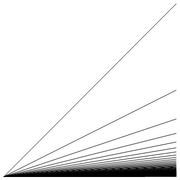9 KB Vipul This file was created using [http://www.wolfram.com/mathematica Wolfram Mathematica 8] with the following code: <pre>Graphics[{Line[{{0, 0}, {1, 0}}], Map[Function[n, Line[{{0, 0}, {1, 1/n}}]], Range]}]</pre> 1
00:02, 21 December 2010 Doublecombspace.png (file)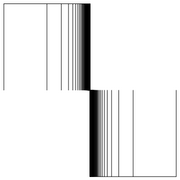2 KB Vipul This file was created using [http://www.wolfram.com/mathematica Wolfram Mathematica 8] with the following code: <pre>Graphics[{Line[{{0, 0}, {1, 0}}], Line[{{0, 0}, {0, 2}}], Line[{{-1, 2}, {0, 2}}], Map[Function[ x, {Line[{{1/x, 0}, {1/x, 1}}] 1
23:59, 20 December 2010 Combspace.png (file)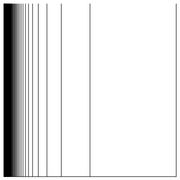2 KB Vipul This file was created using [http://www.wolfram.com/mathematica Wolfram Mathematica 8] with the following code: <pre>Graphics[{Line[{{0, 0}, {1, 0}}], Line[{{0, 0}, {0, 1}}], Map[Function[x, Line[{{1/x, 0}, {1/x, 1}}]], Range]}]</pre> Replacing 1
17:36, 20 December 2010 Homotopyforcompositewithinverse.png (file)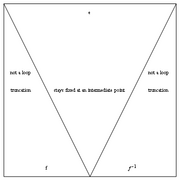4 KB Vipul   3
20:17, 18 December 2010 Homotopyforrightcompositionwithtrivialloop.png (file)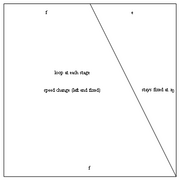3 KB Vipul This file was created using [http://www.wolfram.com/mathematica Wolfram Mathematica 8] with the following code: <pre>Graphics[{Line[{{0, 0}, {0, 1}, {1, 1}, {1, 0}, {0, 0}}], Line[{{1, 0}, {1/2, 1}}], Text["f", {0.5, 0.05}], Text["f", {0.25, 0.95}] 1
19:44, 18 December 2010 Homotopyforleftcompositionwithtrivialloop.png (file)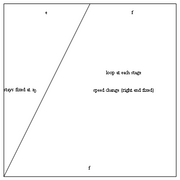3 KB Vipul This file was created using [http://www.wolfram.com/mathematica Wolfram Mathematica 8] with the following code: <pre>Graphics[{Line[{{0, 0}, {0, 1}, {1, 1}, {1, 0}, {0, 0}}], Line[{{0, 0}, {1/2, 1}}], Text["f", {0.5, 0.05}], Text["f", {0.75, 0.95}] 1
19:40, 18 December 2010 Associativityhomotopy.png (file)4 KB Vipul   2
18:43, 18 December 2010 Homotopyofcompositepaths.png (file)2 KB Vipul This file was created using [http://www.wolfram.com/mathematica Wolfram Mathematica 8] with the following code: <pre>Graphics[{Pink, EdgeForm[White], Polygon[{{0.5, 1}, {0.5, 0}, {0, 0}, {0, 1}}], Black, Line[{{0.5, 0}, {0, 0}, {0, 1}, {0.5, 1}}], 1
18:41, 18 December 2010 Homotopyrightofcomposition.png (file)2 KB Vipul This file was created using [http://www.wolfram.com/mathematica Wolfram Mathematica 8] with the following code: <pre>Graphics[{Yellow, EdgeForm[White], Polygon[{{1, 1}, {1, 0}, {0, 0}, {0, 1}}], Black, Line[{{0, 0}, {1, 0}, {1, 1}, {0, 1}}], Dashed 1
18:40, 18 December 2010 Homotopyleftofcomposition.png (file)2 KB Vipul This file was created using [http://www.wolfram.com/mathematica Wolfram Mathematica 8] with the following code: <pre>Graphics[{Pink, EdgeForm[White], Polygon[{{1, 1}, {1, 0}, {0, 0}, {0, 1}}], Black, Line[{{1, 0}, {0, 0}, {0, 1}, {1, 1}}], Dashed, 1
16:48, 11 September 2008 Logo.jpg (file)32 KB Vipul   1